Deutsche Physikalische Gesellschaft

Frühjahrstagung Gravitation und Relativitätstheorie

Jena, 25. Februar - 1. März 2013

GRT - well proven and also incomplete?

J. Brandes

26.2.2013; last update: 21.07.21

Content:

## Introduction

Many thanks for having a talk together with so many professionals.

Incompleteness means some imperfections even contradictions of a theory but which can be overcome by some extension.

## Contradictory results of total energy

The elementary question: What is the total energy EG of a particle resting in the r,t-reference system of Schwarzschild metric (SM)? SM reads:

(1)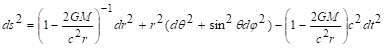Derived from the formulas of free, radial fall one gets , ,  312:

(2)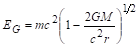This is at least qualitatively correct since removing the particle from the gravitational field needs energy. Doing this the total energy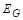of the particle becomes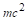and therefore within the gravitational fieldhas to be lower.

On the other side, there is the equivalence principle. A particle resting in its local inertial system (e.g. the free falling particle) has a total energy equal to its rest mass:

(3)Formula (2) and (3) contradict each other. Certainly, they belong to different reference systems with one of them being accelerated, in fact. But: At time point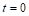the free falling particle is also a resting one within the r,t-reference system since its velocity. Only its acceleration. Special theory of relativity is applicable and therefore the free falling particle atand an always resting particle at the same place possess identical total energies (3). Formula (2) and (3) contradict each other.

On account of the qualitative argument above, formula (2) is the correct one. One can see it again by series expansion of (2):

(4)The second term describes the negative gravitational energy. Approximately formula (2) becomes the rest mass minus Newtonian gravitational energy. Therefore formula (2) meets the Newtonian limit but formula (3) does not.

## Rewording of equivalence principle

On the other side, the equivalence principle is tried and tested. So formula (3) should be correct, too. Rewording this principle a little shows why.

“For measurements within gravitational fields the measuring results within local inertial systems are predicted by special relativity.”

Concerning our application this means: The measurement of EG with measuring instruments resting in the gravitational field yields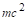. This is no contradiction to (2) any longer if one can assume that measuring instruments become modified by gravitational fields.

## Modification of measuring instruments within gravitational fields

Let us consider possible modifications of measuring instruments during measurement of EG. Let us choose some intellectually simple measuring procedure. Transfer an antiparticle to the resting particle and perform the measurement of annihilation frequency of the two resulting photons. One gets:

(5): Annihilation frequency, measured by a clock resting in the gravitational field: proper time of a clock resting in the gravitational field (-clock, standard clock)

On the other side, it is derivable from SM:

(6)With words. Time passing ofof a t-clock outside the gravitational field means time passing of a-clock less by a factor

(7)Therefore, the measured frequencies are:

(8)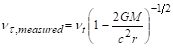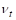: ‘real’ frequency, slowing down of clocks by gravitational fields eliminated.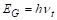: ‘real’ energy

(8) inserted into (5) yields, identical with formula (2).

With words: Taking into account the modification of measuring instruments by gravitational field – in this case the slowing down of clocks – makes it possible to derive the total energy of a resting particle by use of the equivalence principle. The contradiction of (2) and (3) is solved.

## More about modification of measuring instruments within gravitational fields

What does formula (6) mean?

(6)Within GRT this is interpreted as: ‘time is curved’ or ‘time runs slower within the gravitational field than outside of it’. Lorentz interpretation of GRT (LI of GRT) says: ‘standard clocks are slowed down inside the gravitational field’ .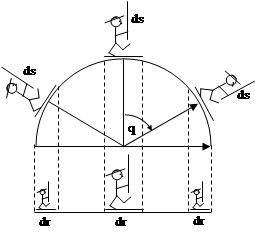The picture illustrates this for measuring rods. Within gravitational fields one can argue - as GRT does: measuring rods don’t change but space is curved or – as LI of GRT does - space remains flat but measuring rods contract .

## Objections by V. Perlick

V. Perlick wrote: „... [Die obige] Argumentation laeuft immer auf dieselbe Be­hauptung hinaus:
„Das Problem für die Klassische Allgemeine Relati­vitätstheorie ist die Entscheidung, welches Messer­gebnis die Energie eines im Gravitationsfeld ruhen­den Teilchens wiedergibt und welches umgerechnet werden muss. Beides zugleich ist falsch.“
Da widerspreche ich entschieden: Beides ist richtig.
Es handelt sich eben in einem Fall um die Energie in Bezug auf die eine Uhr, im anderen Fall in Bezug auf die andere Uhr. Beide Uhren sind wohldefiniert und wohlverstanden, und die Umrechnungsformel ist, wie Sie richtig schreiben, auch wohlbekannt. Von einer 'Unvollstaendigkeit' der Theorie kann deshalb keine Rede sein. Eine Paradoxie laege frei­lich vor, wenn die Situation gegenueber Vertau­schung der beiden Uhren symmetrisch waere. Das ist aber natuerlich nicht der Fall, da ja nur eine der beiden Uhren eine Standarduhr ist.“
The main argument of V. Perlick, as far as I understand:
Formula (3) is correct since it is proved by standard clocks and formula (2) is correct as well, since it is the same as formula (3) but using t-clocks. Start with the measurement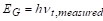and then convert with formula (8) into the ‘correct’ standard clock time,and by use of formula (5) insertinstead of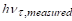getting formula (2).

Everything depends on the clock you are measuring with.

What is not correct? The measuring result is not the true result if clocks run slow or fast. Following LI of GRT you can say that t-clocks measure the correct time since standard clocks are slowed down in gravitational fields or following GRT that instead standard clocks measure correct time and t-clocks do not but you cannot have both of the statements together. Only formula (2) or (3) is the correct formula.

## Summary

Classical general theory of relativity knows two formulas of total energy of a particle resting within gravitational fields contradicting each other. This contradiction is resolved if one can assume that measuring instruments become modified by gravitational fields. This is LI of GRT.

Many thanks to V. Perlick for our discussion.

Literature

 Schutz, B. (2003): Gravity from the ground up. Cambridge University Press, see p 232ff. Formula (2) is evaluated but with  restricted range of validity.

 Brandes, J.; Czerniawski, J. (2010): Spezielle und Allgemeine Relativitätstheorie für Physiker und Philosophen – Einstein- und Lorentz-Inter­pretation, Paradoxien, Raum und Zeit, Experi­mente. VRI,
4. erweiterte Auflage, 404 Seiten, 100 Abbil­dungen, ISBN 978-3-930879-08-3 Näheres (Preis, Inhaltsverzeichnis etc.): http://www.buchhandel.de/ oder http://www.amazon.de/ Suchen mit 9783930879083

 Dragon, Norbert (2012): Geometrie der Relativitätstheorie. http://www.itp.uni-hannover.de/~dragon. pdf-file on homepage of the author. There equation (6.24) with dr/dtau = 0 and L=0 results in formula (2) without  restricted range of validity.

home